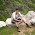## January 04, 2014

### Problem: Energy Consumption

I came across an interesting problem and thought I would share it with you folks:

A battery-operated 65nm digital CMOS device is found to consume equal amounts (P) of dynamic power and leakage power while the short-circuit power is negligible. The energy consumed by a computing task, that takes T seconds, is 2PT.

Compare two power reduction strategies for extending the battery life:
• Clock frequency is reduced to half, keeping all other parameters constant.
• Supply voltage is reduced to half. This slows the gates down and forces the clock frequency to be lowered to half of its original (full voltage) value. Assume that leakage current is held unchanged by modifying the design of transistors.
Please post your answers as comments to this blog post.

Source: Dynamic and Static Power in CMOS. A Presentation by Vishwani D. Agrawal, Auburn University, USA and Srivaths Ravi,Texas Instruments India.

#### 15 comments:

1.Leakage power is independent of switching and hence in the first case energy reduces to 1.5PT while in second case leakage power reduces by half and dynamic power reduces to 1/8th. However this assumes the computing task still needs the same time T even with reduced frequency. Do we need to consider that it will take time 2T? Then problem gets more interesting!

2.Hi Shri!

Yes! That was the main catch! The computing time needs to be taken into account because the problem asks the energy and not the power. I am sure you'll get it right in the second attempt. I'd be looking forward to your answer. :)

Thanks!
Naman

1.Hi Naman,

OK in that case the energy consumed is 3PT for first case and 2(PT/8 + PT/2) = 1.25PT for the second case. Having said that, is it fair to assume leakage power to be same as dynamic power?

--
Shrikant

2.That's the right answer and you made an interesting point.

While leakage power would depend on the input combination, the dynamic power would depend on the frequency. I have worked on 65 and 45nm technology nodes, and I have observed that dynamic power of a circuit (like an 8-bit synchronous counter) at 20 Meg is ~10 times the leakage power of the same circuit.

With shrinking technology node, the speed of gates is increasing and the target frequency is going up, however the leakage power is also increasing exponentially. However, for low to medium frequencies (kHz-1Meg), my bet would be that both leakage and dynamic power would be pretty much comparable. I'll try and get back to you with some concrete data.

Thank you so much for posting the answer!

Regards,
Naman

3.Pleasure to have got it right.! Yes leakage power is definitely a serious concern with technology scaling.
It will be interesting to have a look at your numbers, waiting for that.

Thanks,
Shrikant

3.This comment has been removed by the author.

4.So in the first case there is no energy saving as the same task will require time 2T. But in the second case you will witness 25% reduction in energy. But in a practical scenario voltage and frequency are varied based on the task at hand and this will yield better energy savings.

1.Hi Kiran,

I am sorry but your answer is incorrect. However, you made an interesting point that in a practical scenario, the voltage and frequency are varied based on the task at hand. Could you please elaborate a little on it?

I would urge you to give another try to the problem.

Thanks!

Regards,
Naman

2.Great, Shri has got it right.:-)
The point i was trying to make was, say in the case of mobiles when in standby mode, device is put into deep sleep state, where voltage and frequency are reduced to the lowest possible extent and when you play angry birds, both get bumped up. Thus in a practical scenario a task is mapped to one set of voltage and frequency so waiting for time 2T to finish the same task might not be preferable. Please let me know if i have missed something.

3.Hi Naman,

I agree with Shri's answer about the second scenario but for the first one, I think power increases to 3PT. Assuming the total power 2PT of the device from equal amounts of dynamic (PT) as well as leakage power (PT). the dynamic power remains unchanged as P reduces to P/2 and time of operation increases to 2T. Coming to leakage power, the part of the circuit that contributed for leakage will keep leaking for 2T amount of time and that gives 2PT in energy contribution(leakage power is unchanged though). so the total would be 3PT. Let me know if my assumptions are incorrect.

Thanks!

4.Hi Karthikeya,

Your assumptions are correct. Thanks for posting the answer.

Regards,
Naman

5.I truly like to reading your post. Thank you so much for taking the time to share such a nice information.solar power

6.When the supply voltage and clock frequency are reduced to half their values, dynamic power is reduced to P / 8 and static power to P / 2. The time of task is doubled and the total energy consumption is,
Energy = (P / 8 + P / 2) 2T = 5PT / 4 =1.25PT
The voltage reduction strategy reduces energy consumption while a simple frequency reduction consumes more energy.

7.Awesome ! i really found very informative article here and bookmarked this blog. Thank you.How Solar Panels work

8.Can you please post the dynamic and leakage power equations?